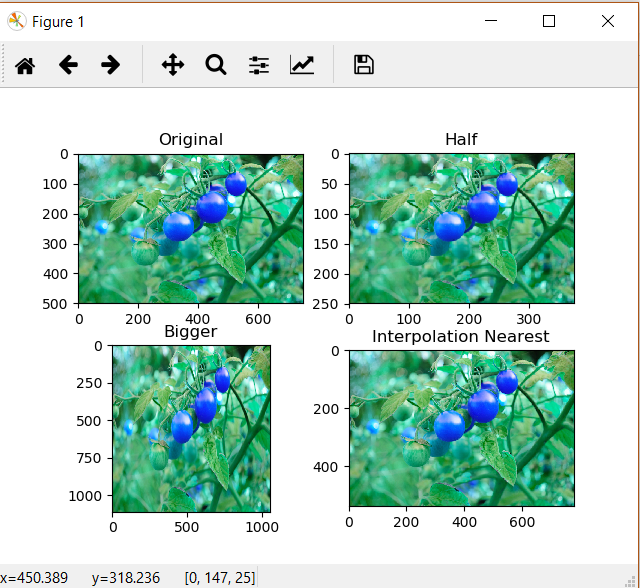Image Resizing using OpenCV | Python

• Difficulty Level : Easy
• Last Updated : 15 Mar, 2021

Image resizing refers to the scaling of images. Scaling comes in handy in many image processing as well as machine learning applications. It helps in reducing the number of pixels from an image and that has several advantages e.g. It can reduce the time of training of a neural network as more is the number of pixels in an image more is the number of input nodes that in turn increases the complexity of the model.
It also helps in zooming in images. Many times we need to resize the image i.e. either shrink it or scale up to meet the size requirements. OpenCV provides us several interpolation methods for resizing an image.

Choice of Interpolation Method for Resizing –

• cv2.INTER_AREA: This is used when we need to shrink an image.
• cv2.INTER_CUBIC: This is slow but more efficient.
• cv2.INTER_LINEAR: This is primarily used when zooming is required. This is the default interpolation technique in OpenCV.

Below is the code for resizing.

Python3

 import cv2 import numpy as np import matplotlib.pyplot as plt   image = cv2.imread("C://gfg//tomatoes.jpg", 1) # Loading the image   half = cv2.resize(image, (0, 0), fx = 0.1, fy = 0.1) bigger = cv2.resize(image, (1050, 1610))   stretch_near = cv2.resize(image, (780, 540),                interpolation = cv2.INTER_NEAREST)     Titles =["Original", "Half", "Bigger", "Interpolation Nearest"] images =[image, half, bigger, stretch_near] count = 4   for i in range(count):     plt.subplot(2, 2, i + 1)     plt.title(Titles[i])     plt.imshow(images[i])   plt.show()

Output:Note: One thing to keep in mind while using the cv2.resize() function is that the tuple passed for determining the size of the new image ((1050, 1610) in this case) follows the order (width, height) unlike as expected (height, width).

My Personal Notes arrow_drop_up
Recommended Articles
Page :